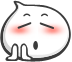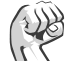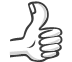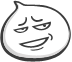### Aspose.Cells使用模板

```//模板文件路径
string Template_File_Path = @".\Template\Template.xlsx";
//  打开 Excel 模板
Workbook CurrentWorkbook = File.Exists(Template_File_Path) ? new Workbook(Template_File_Path) : new Workbook();
//  打开第一个sheet
Worksheet sheet = CurrentWorkbook.Worksheets;
// 比如要在 A1 位置写入 Demo这个值
Cell itemCell = sheet.Cells["A1"];
itemCell.PutValue("Demo");
//  获取 Table 数据
DataTable dt = GetData();            //  写入数据的起始位置
string cell_start_region = "C1";
//  获得开始位置的行号
int startRow = sheet.Cells[cell_start_region].Row;
//  获得开始位置的列号
int startColumn = sheet.Cells[cell_start_region].Column;  //  写入Excel。参数说明，直接查阅文章底部文档链接
sheet.Cells.ImportDataTable(dt, false, startRow, startColumn, true, true);
//  设置执行公式计算 - 如果代码中用到公式，需要设置计算公式，导出的报表中，公式才会自动计算
CurrentWorkbook.CalculateFormula(true);
//  生成的文件名称
string ReportFileName = string.Format("Excel_{0}.xlsx", DateTime.Now.ToString("yyyy-MM-dd"));
//  保存文件
CurrentWorkbook.Save(@".\Excel\" + ReportFileName, SaveFormat.Xlsx);```

关键字：### 鼓掌

0### 正能量

0### 赞

0### 呵呵

0

##### 企业级大促